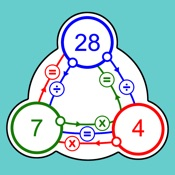# Super Number Triangles - Lumos Educational App Store1
Price -Free
\$0

#### DESCRIPTION:

Super number triangles is a visual maths game for children to help them learn their times tables in both directions from 2-12 (multiplication and division). For those that already know their times tables, the highest game level is a 'Sudoku' logic style game using all times tables knowledge. The early stages of learning basic maths skills can make a big difference on whether your child finds maths a chore or a joy. Sometimes presenting something differently can just make everything click into place. Times tables are one of the elements of maths that many children learn through memory

#### OVERVIEW:

Super Number Triangles is a free educational mobile app By Fabula Ltd.It helps students in grades 3,4,5 practice the following standards 3.OA.7,4.NBT.5,5.NBT.2.

This page not only allows students and teachers download Super Number Triangles but also find engaging Sample Questions, Videos, Pins, Worksheets, Books related to the following topics.

1. 3.OA.7 : Fluently multiply and divide within 100, using strategies such as the relationship between multiplication and division (e.g., knowing that 8 .

2. 4.NBT.5 : Multiply a whole number of up to four digits by a one-digit whole number, and multiply two two-digit numbers, using strategies based on place value and the properties of operations. Illustrate and explain the calculation by using equations, rectangular arrays, and/or area models. (Grade 4 expectations in this domain are limited to whole numbers less than or equal to 1,000,000. A range of algorithms may be used.).

3. 5.NBT.2 : Explain patterns in the number of zeros of the product when multiplying a number by powers of 10, and explain patterns in the placement of the decimal point when a decimal is multiplied or divided by a power of 10. Use whole number exponents to denote powers of 10..

3
4
5

#### STANDARDS:

3.OA.7
4.NBT.5
5.NBT.2

Developer: Fabula Ltd

Software Version: 1.01

Category: Education

### RELATED APPSEdSearch WebSearch